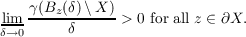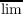#### Vol. 70, No. 2, 1977

 Recent Issues Vol. 323: 1 Vol. 322: 1  2 Vol. 321: 1  2 Vol. 320: 1  2 Vol. 319: 1  2 Vol. 318: 1  2 Vol. 317: 1  2 Vol. 316: 1  2Online Archive Volume: Issue:The Journal Subscriptions Editorial Board Officers Contacts Submission Guidelines Submission Form Policies for Authors ISSN: 1945-5844 (e-only) ISSN: 0030-8730 (print) Special Issues Author Index To Appear Other MSP Journals
Rational approximation and the growth of analytic capacity

### Claes Fernström

Vol. 70 (1977), No. 2, 347–350
##### Abstract

Let X be a compact set in the complex plane C. Denote by R(X) the closure in the supremum norm of the rational functions with poles off X and by A(X) the set of continuous functions, which are analytic on the interior of X. The analytic capacity of a set S is denoted by γ(S). For the definition of γ see below. Let Bz(δ) = {ζ C;|z ζ| < δ} and let ∂X denote the boundary of X. Vitushkin has proved that R(X) = A(X) ifLet ψ be a function from R+ to R+, where R+ = {x R;x 0}. We now ask the following questions. If limδ0ψ(δ) = 0, is it possible to find a compact set X such that R(X)A(X) and such that γ(Bz(δ) X) δψ(δ) for all z ∂X and for all δ, 0 < δ < δz? If the answer is yes, can the answer still be yes, if limδ0ψ(δ) = 0 is replaced byδ0ψ(δ) > 0? The answers of these questions can be found in Theorem 1 and Theorem 2.

##### Mathematical Subject Classification
Primary: 30A82, 30A82
##### Milestones